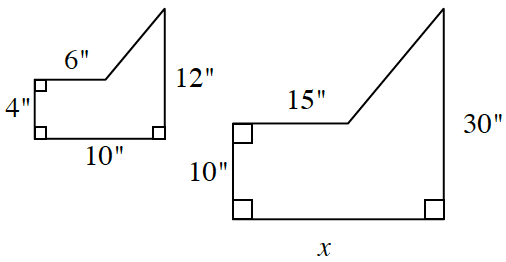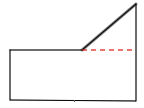### Home > INT2 > Chapter 4 > Lesson 4.1.4 > Problem4-41

4-41.The two polygons at right are similar.

1. Determine the value of $x$. Show all work.

Set up a proportion to determine the value of $x$.

2. Calculate the area of each polygon.

Divide each polygon into smaller, easier polygons, then calculate the areas.area of smaller figure: $56$ in²
area of larger figure: $350$ in²